#### 期刊菜单

Study on the Combustion Characteristics of the Circulating Fluidized Bed Boiler Influenced by Air Volume Ratios
DOI: 10.12677/MOS.2021.104091, PDF, HTML, XML, 下载: 187  浏览: 277  科研立项经费支持

Abstract: Circulating fluidized bed (CFB) boilers have been widely used due to the high combustion efficiency, good fuel adaptability and low pollutant emission. This paper conducted a numerical simulation analysis on a 240 t/h circulating fluidized bed boiler to analyze the influence of changing the ratio of primary and secondary air on the internal flow and combustion characteristics. The results show that the small proportion of primary air θ resulted in poor effect of fluidization inside the furnace; too large proportion of primary air θ cannot provide oxygen for the combustion in the center of the furnace timely; when the proportion of primary air θ is 0.55 (Condition III), the bed material and temperature distributions are even, and NOx concentration at the outlet is the lowest.

1. 引言

2. 锅炉概况(a) 炉膛三维模型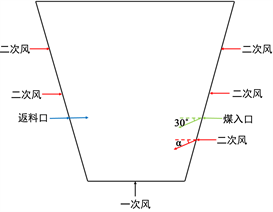(b) 密相区炉膛截面图

Figure 1. Furnace physical model diagram

3. 数学模型及参数设置

3.1. 数学模型

1) 挥发分析出的反应式为：

$\text{Volatile}〈\text{s}〉⇒\text{CO}+{\text{CO}}_{2}+{\text{H}}_{\text{2}}\text{O}+\text{tar}$ (1)

$prod=yi\ast \text{C_R}/mw$ (2)

$\text{*}rr=\text{0}\text{.01}\text{\hspace{0.17em}}\text{Arrhenius_devolatilization}*{e}^{\left(E/\left(8.314*T\right)\right)}*prod*\text{C_VOF}$ (3)

2) 焦炭燃烧反应式为：

$\text{C}〈\text{s}〉+{\text{O}}_{2}⇒\text{CO}$ (4)

UDF中涉及的计算有脱附速率(Rd)、扩散系数(diff)、雷诺数(Re)、正反应速率系数(k_f)、逆反应速率系数(k_r)以及中间变量(rr')，各计算过程不再赘述，最终反应速率公式  为：

$*rr=\frac{rr\text{'}\text{\hspace{0.17em}}*p\text{_}o2*6*C\text{_}VOF}{D\text{_}p*3200}$ (5)

3.2. 边界条件设置Table 1. Circumstances of different air volumes

4. 结果与讨论

4.1. 流动特性研究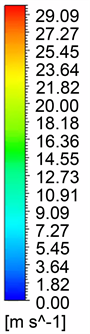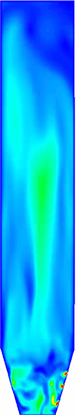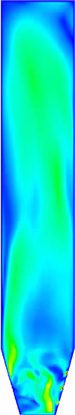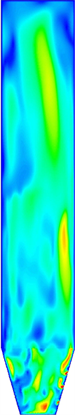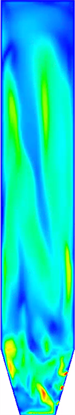工况一(θ = 0.45) 工况二(θ = 0.5) 工况三(θ = 0.55) 工况四(θ = 0.6) 工况五(θ = 0.65)

Figure 2. Vapor velocity distribution of X = 0 section under different conditions工况一(θ = 0.45) 工况二(θ = 0.5) 工况三(θ = 0.55) 工况四(θ = 0.6) 工况五(θ = 0.65)

Figure 3. Vapor velocity distribution of X = 0 section under different conditions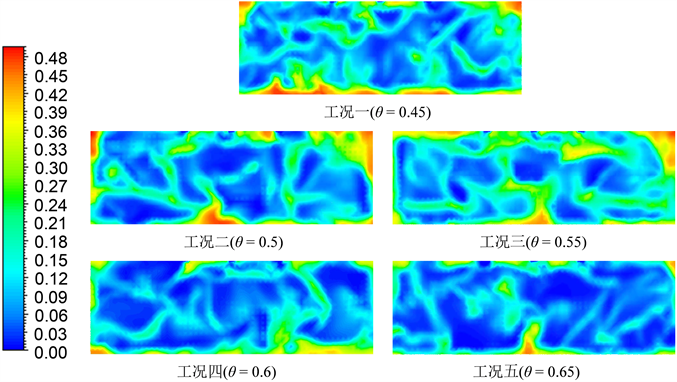Figure 4. Distribution of bed material volume fraction of Z = 1 m section under different conditions

4.2. 温度场分布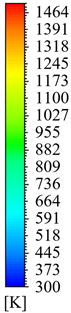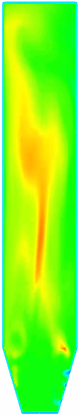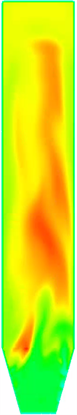工况一(θ = 0.45) 工况二(θ = 0.5) 工况三(θ = 0.55) 工况四(θ = 0.6) 工况五(θ = 0.65)

Figure 5. Temperature distribution of X = 0 section under different conditions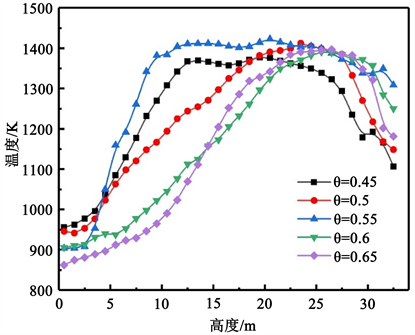Figure 6. Average temperature change curve in Z direction under different working conditions

4.3. NOx质量浓度分析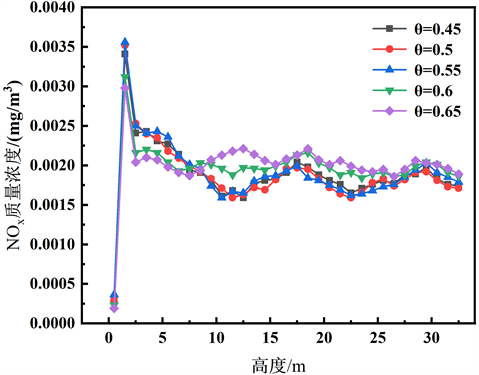Figure 7. Variation curve of average mass concentration of NOx in Z direction under different conditions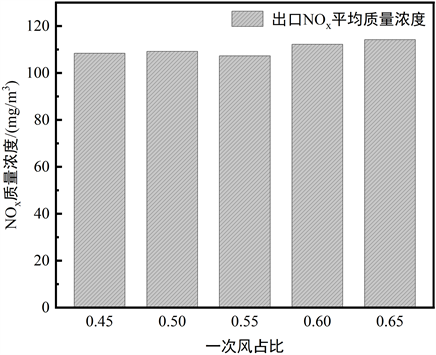Figure 8. Export NOx average mass concentration under different conditions

5. 结论

1) 在流动过程中，一次风起到流化炉膛内部各物料的作用，二次风起到增强炉膛内部的扰动作用；在燃烧过程中，一次风在决定燃料炉内停留时间中起主要作用，二次风主要起到补充燃烧的作用。

2) θ过小，对炉膛内部流化效果差，不利于密相区的气固混合；θ过大，此时二次风风速较低，难以为炉膛中心提供足量的氧气辅助燃烧。

3) 模拟结果显示，当θ = 0.55时，炉膛内部床料、温度分布均匀，炉膛中部出现较大的高温区域，且出口NOx质量浓度低，为最佳配风方案。

NOTES

*通讯作者。

  徐旭常, 吕俊复, 张海. 燃烧理论与燃烧设备[M]. 第2版. 北京: 科学出版社, 2012.  姚禹歌, 黄中, 张缦, 杨海瑞, 吕俊复, 岳光溪. 中国循环流化床燃烧技术的发展与展望[J/OL]. 热力发电: 1-8[2021-09-23]. http://kns.cnki.net/kcms/detail/61.1111.TM.20210528.1721.002.html  刘雪敏, 尹炜迪, 汪佩宁, 杨海瑞. 循环流化床锅炉床压降对其燃烧效率的影响[J]. 煤炭学报, 2016, 41(10): 2484-2489.  Liu, Z., Zhong, W., Shao, Y. and Liu, X. (2020) Exergy Analysis of Supercritical CO2 Coal-Fired Circulating Fluidized Bed Boiler System Based on the Combustion Process. Energy, 208, 118327. https://doi.org/10.1016/j.energy.2020.118327  苗苗, 张缦, 吕俊复, 杨海瑞, 张凯. 流化床燃烧中N_2O生成机理与减排技术[J]. 清华大学学报(自然科学版), 2020, 60(6): 507-517.  Li, J.-J., Zhang, M., Yang, H.-R., Lu, J.-F., Zhao, X.-X. and Zhang, J.-C. (2016) The Theory and Practice of NO Emission Control for Circulating Fluid-ized Bed Boilers Based on the Re-Specification of the Fluidization State. Fuel Processing Technology, 150, 88-93. https://doi.org/10.1016/j.fuproc.2016.05.004  肖卓楠, 徐鸿, 陈伟鹏, 张智羽. CFB锅炉采用富氧燃烧与常规燃烧方式下的热经济性分析对比[J]. 锅炉技术, 2019, 50(1): 26-31.  张奇月, 颜勇, 段元强, 段伦博. 超超临界350 MW富氧燃烧循环流化床设计[J]. 热力发电, 2020, 49(5): 73-80.  柯希玮, 张缦, 杨海瑞, 吕俊复, 郭学茂, 李军, 等. 循环流化床锅炉NO_(x)生成和排放特性研究进展[J]. 中国电机工程学报, 2021, 41 (8): 2757-2771.  孙经雷. 浅谈循环流化床锅炉一次风与二次风的使用[J]. 煤炭科技, 2016(4): 64-66.  马有福, 王凡, 袁益超, 吕俊复, 王子睿. 循环流化床锅炉一次风布风均匀性数值研究[J]. 锅炉技术, 2018, 49(6): 35-41.  杨雪芬, 鲁佳易, 薛大勇, 韦立校. 基于CPFD模型的循环流化床二次风穿透特性研究[J]. 机电信息, 2020(35): 22-24.  官伟. 90 t/h循环流化床锅炉节能改造优化方案[J]. 中国设备工程, 2020(8): 81-82.  彭丹, 王贲, 李威, 许超. 基于空气分级的循环流化床锅炉低氮燃烧模拟[J]. 锅炉技术, 2020, 51(5): 30-36.  Syamlal, M. and Wittmann, C.V. (1985) Continuous Reaction Mixture Model for Coal Liquefaction Kinet-ics. Industrial & Engineering Chemistry Fundamentals, 24, 82-90. https://doi.org/10.1021/i100017a014